Flat

If a function has the Flat attribute nested layers are automatically flattened during evaluation.  This aspect of how Flat works is understood by many Mathematica users, and is demonstrated in the cell below.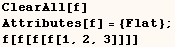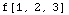I was only able to understanding many of the subtle ways that Flat works after getting Technical Support from Dave Withoff.  One subtle point many users don't understand is the effect the Flat attribute has on pattern matching.  In the cell below (f) still has the Flat attribute and no other attributes.  In the example below the pattern matcher tries to find a way to make the expression match the pattern.  The pattern matcher finds that the pattern matches when the f[1,2,3,4] is treated as f[f,f[2,3,4]] and the two are considered equivalent to when (f) has the Flat attribute.  When the definition is applied f[f,f[2,3,4]] evaluates to {f,f[2,3,4]}.  Then f[2,3,4] is treated as f[f,f[3,4]] which evaluates to {f,f[3,4]}.  Then f[3,4] evaluates to {f,f}.  When this is all put together we get   (  {f{f{f,f}}}  ).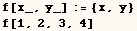The result above would be different if (f) had the attributes Flat and OneIdentity.  For further discussion of this see the details of OneIdentity, Flat.

A warning about attributes and pattern matching

The attributes Flat, Orderless, and OneIdentity effect pattern matching.  If a user defined function needs either of these attributes, they should be set before any definitions are entered, and not changed after the definitions are made.  This way the given attributes effects both pattern matching and the evaluation process (usually the desired effect).  This point is demonstrated for the Flat attribute in the cells below.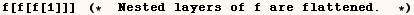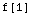If definition(s) are given before the attributes are set then the attribute effects evaluation, but not pattern matching.  This is demonstrated in the next few cells.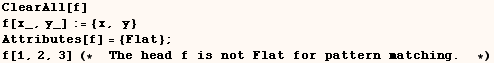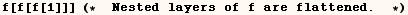When the next input cell is evaluated (f) is Flat for pattern matching, but not for evaluation.

A problem with the Flat attribute and how to solve it

A Wolfram Research Technical Support web page points out that one should avoid definitions such as (f[p_]:=p) for functions that have the Flat attribute. In the cell below this was attempted,and the kernel would have gone into infinite iteration if it didn't bail out when the iteration limit was exceeded. The reason this leads to infinite iteration is that the pattern matcher tries to rewrite f[1,2] so (f) has only one argument so the expression will match the pattern (f[p_]). The pattern matcher is able to fit the expression to the pattern by treating f[1,2] as f[f[1,2]].The outer (f) is evaluated and results in f[1,2] which has to be evaluated.However,this is exactly where we started. Then the kernel does this over and over until the iteration limit is exceeded.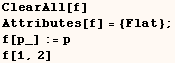Plus and Times have the Flat attribute. At first glance it seems Plus and Times have the definitions
( Plus[p_]:=p; Times[p_]:=p ) but it isn't that simple. Something special had to be done because Plus[a,b] and Times[a,b] don't cause an \$IterationLimit message. Allan Hayes indicated the following definition can be used to allow a user defined function to work like Plus and Times in this regard. I suspect this works because the definition for f[a__]  is only used after pattern matching is finished.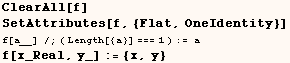In the next output cell we see nested layers of (f) are flattened.  In addition the result is f[1,2] which does not lead to infinite iteration.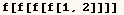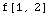Next we see (f) is flat for pattern matching. In this case f[1.5, 2.5, 3.5] was treated as the equivalent expression f[1.5,f[2.5,3.5]] which evaluated to {1.5, {2.5, 3.5}}.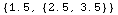Finally we verify that when (f) is evaluated with only one argument it returns the argument.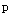Created by Mathematica  (May 16, 2004)

Back to Ted’s Tricks index page